{{ message }}

# replace parse() with parse_safe() in geom_text()#2867

Merged
merged 19 commits into from Sep 4, 2018
Merged

# replace parse() with parse_safe() in geom_text()#2867

merged 19 commits into from Sep 4, 2018

## Conversation

###slowkow commented Aug 28, 2018

 The built in `parse()` function silently drops items: ``````length(parse(text = c("alpha", "", "gamma"))) `````` We expect the length to be 3, but instead it is 2. So, we add a new function `parse_safe()` that keeps all items. This addresses issue #2864 The text was updated successfully, but these errors were encountered:
We are unable to convert the task to an issue at this time. Please try again.
``` use parse_safe() in geom_text() ```
``` 34c01cc ```
```The built in `parse()` function silently drops items:

length(parse(text = c("alpha", "", "gamma")))

We expect the length to be 3, but instead it is 2.

So, we add a new function `parse_safe()` that keeps all items.

###slowkow commented Aug 28, 2018 • edited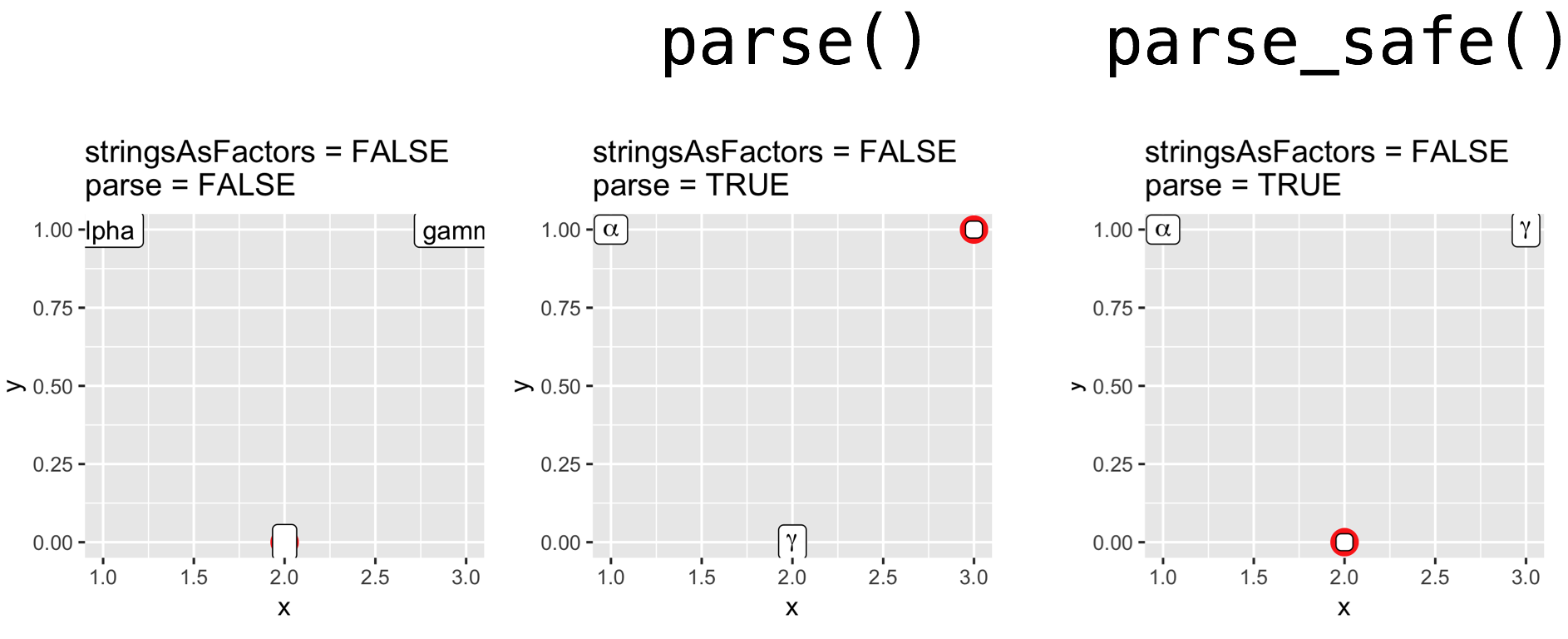# Details

Here is the code I used to generate the images.

```dev.off()
library(ggplot2)
library(patchwork)

d1 <- data.frame(
x = c(1, 2, 3),
y = c(1, 0, 1),
label = c("alpha", " ", "gamma"),
stringsAsFactors = FALSE
)
p <- ggplot(d1, aes(x = x, y = y, label = label)) + geom_point(size = 5, color = "red")
p1 <- p + geom_text() + labs(title = "stringsAsFactors = FALSE\nparse = FALSE")
p2 <- p + geom_text(parse = TRUE) + labs(title = "stringsAsFactors = FALSE\nparse = TRUE")
p1 + p2
ggsave(filename = "pullXXX/d1-text.png", plot = p1 + p2, width = 6, height = 3)

p1 <- p + geom_label() + labs(title = "stringsAsFactors = FALSE\nparse = FALSE")
p2 <- p + geom_label(parse = TRUE) + labs(title = "stringsAsFactors = FALSE\nparse = TRUE")
p1 + p2
ggsave(filename = "pullXXX/d1-label.png", plot = p1 + p2, width = 6, height = 3)

d2 <- data.frame(
x = c(1, 2, 3),
y = c(1, 0, 1),
label = c("alpha", " ", "gamma"),
stringsAsFactors = TRUE
)
p <- ggplot(d2, aes(x = x, y = y, label = label)) + geom_point(size = 5, color = "red")
p1 <- p + geom_text() + labs(title = "stringsAsFactors = TRUE\nparse = FALSE")
p2 <- p + geom_text(parse = TRUE) + labs(title = "stringsAsFactors = TRUE\nparse = TRUE")
p1 + p2
ggsave(filename = "pullXXX/d2-text.png", plot = p1 + p2, width = 6, height = 3)

p1 <- p + geom_label() + labs(title = "stringsAsFactors = TRUE\nparse = FALSE")
p2 <- p + geom_label(parse = TRUE) + labs(title = "stringsAsFactors = TRUE\nparse = TRUE")
p1 + p2
ggsave(filename = "pullXXX/d2-label.png", plot = p1 + p2, width = 6, height = 3)```

###clauswilke commented Aug 29, 2018

 Several comments: This still parses twice. It must be possible to parse only once, even if the result should be an expression object and not a list. Please set `keep.source = FALSE` in `parse()`: `parse(text = text, keep.source = FALSE)` Otherwise regression tests won't work. Please add a few regression tests, including testing with character vectors, factors, data with `NA`s, and data containing empty strings. They will generally look like this (in the testthat suite): `expect_equal(parse_safe(c("A", "B", "C")), expression(A, B, C))`

###clauswilke commented Aug 29, 2018 • edited

 Does this version of Hadley's original function do what you need? [Though I still don't understand why a list of expressions won't work. It does in my testing with `textGrob()` (see here.)] ```parse_hadley <- function(text) { text <- as.character(text) out <- vector("list", length(text)) for (i in seq_along(text)) { expr <- parse(text = text[[i]]) out[[i]] <- if (length(expr) == 0) "" else expr[] } do.call(expression, out) }```

###hadley commented Aug 29, 2018

 I think `vector("expression", length(text))` is slightly preferential than the final `do.call()` (partly because the semantics are so complex when the inputs are expressions)

###slowkow commented Aug 29, 2018

 Claus: This pull request was created before our nice discussion in #2864. It needs to be updated. Regarding each of your comments: I will change the `parse_safe()` function to use this code from the discussion in issue #2864: ```parse_hadley2 <- function(text) { out <- vector("expression", length(text)) for (i in seq_along(text)) { expr <- parse(text = text[[i]], keep.source = FALSE) out[[i]] <- if (length(expr) == 0) NA else expr[] } out }``` Will do! I agree that tests are crucial, will do! I still don't understand why a list of expressions won't work Your code appears to work when you test it with `c("a", "", "b")`: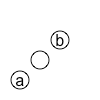But it fails when you test it with `c("alpha", "", "gamma")`: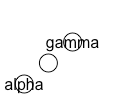###clauswilke commented Aug 29, 2018 • edited

I've figured out why lists of expressions don't work here but seem to work in other parts of ggplot2. We're actually converting them back to expression objects, e.g. here:

Lines 35 to 41 in 71cb174

 if (is.list(labels)) { if (any(sapply(labels, is.language))) { labels <- do.call(expression, labels) } else { labels <- unlist(labels) } }

It's good to keep that in mind, as I think the ggplot2 code is a bit inconsistent in this regard. For example, my original code was inspired by this line:

Line 481 in 34c01cc

 graticule\$degree_label <- lapply(graticule\$degree_label, function(x) parse(text = x)[])

Also, functions in the scales package return lists of expressions rather than expression objects:

```> scales::math_format()(1:5)
[]
10^`1`

[]
10^`2`

[]
10^`3`

[]
10^`4`

[]
10^`5````

In summary: I agree with your latest approach to `parse_safe()`, which returns an expression object. I'm wondering to what extent other parts of the code should use this function also, e.g. the code in sf I linked to above.

added 3 commits Aug 30, 2018
``` update parse_safe() ```
``` fe7216a ```
```Use Hadley's code to parse the expressions once instead of twice.

Use `parse(..., keep.source = FALSE)`, as Claus recommended.

Add an example to demonstrate why `parse_safe()` is needed.```
``` add tests for parse_safe() ```
``` aa9bfc3 ```
``` add note about parse(text = NULL) ```
``` 5dd869e ```

###slowkow commented Aug 30, 2018

 I agree that there might be other places, in addition to `geom-text.r` and `geom-label.r` that could benefit from the new `parse_safe()` function. It would be nice to demonstrate that they suffer from the same problem before I modify them. Please share if you have any examples that demonstrate the issue!

###clauswilke commented Aug 30, 2018

Kamil, here you go. I assume it's caused by this line:

Line 481 in 34c01cc

 graticule\$degree_label <- lapply(graticule\$degree_label, function(x) parse(text = x)[])

Reprex:

```library(ggplot2)
nc <- sf::st_read(system.file("shape/nc.shp", package = "sf"), quiet = TRUE)

# works
ggplot(nc) +
geom_sf(aes(fill = AREA)) +
scale_y_continuous(
breaks = c(34, 35, 36),
labels = c("34*degree*N", "35*degree*N", "36*degree*N")
)``````# breaks
ggplot(nc) +
geom_sf(aes(fill = AREA)) +
scale_y_continuous(
breaks = c(34, 35, 36),
labels = c("34*degree*N", "", "36*degree*N")
)
#> Error in parse(text = x)[]: subscript out of bounds```

Created on 2018-08-30 by the reprex package (v0.2.0).

added a commit to slowkow/ggplot2 that referenced this issue Aug 30, 2018
``` use parse_safe() in sf.R ```
``` a52e7fd ```
```This patch fixes an example that triggers an error:

library(ggplot2)
nc <- sf::st_read(system.file("shape/nc.shp", package = "sf"), quiet = TRUE)
ggplot(nc) +
geom_sf(aes(fill = AREA)) +
scale_y_continuous(
breaks = c(34, 35, 36),
labels = c("34*degree*N", "", "36*degree*N")
)
#> Error in parse(text = x)[]: subscript out of bounds

See tidyverse#2867 for more details.```

###slowkow commented Aug 30, 2018

 Thanks, Claus. My commit a52e7fd fixes the `geom_sf()` issue.

###slowkow commented Aug 30, 2018 • edited

Here is another example of this issue (the alpha should be on the first facet):

```library(ggplot2)
mtcars\$cyl2 <- factor(mtcars\$cyl, labels = c("alpha", "beta", "gamma"))
mtcars\$cyl2 <- as.character(mtcars\$cyl2)
mtcars\$cyl2[mtcars\$cyl2 == "beta"] <- ""
p <- ggplot(mtcars, aes(wt, mpg)) + geom_point()
p + facet_grid(. ~ cyl2, labeller = label_parsed)```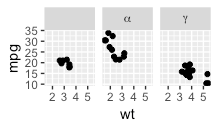Here's the relevant code:

Lines 152 to 169 in 510b4b4

 #' @rdname labellers #' @export label_parsed <- function(labels, multi_line = TRUE) { labels <- label_value(labels, multi_line = multi_line) if (multi_line) { # Using unname() and c() to return a cleaner and easily testable # object structure lapply(unname(labels), lapply, function(values) { c(parse(text = as.character(values))) }) } else { lapply(labels, function(values) { values <- paste0("list(", values, ")") lapply(values, function(expr) c(parse(text = expr))) }) } } class(label_parsed) <- c("function", "labeller")

It turns out I'm wrong. The example works just fine! The "beta" data points are displayed before the "alpha" data points, so it is ok.

To see that everything is ok, compare this figure to the previous one:

`p + facet_grid(. ~ cyl, labeller = label_parsed)`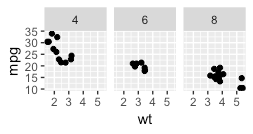``` use parse_safe() in sf.R ```
``` 252e7b2 ```
```This patch fixes an example that triggers an error:

library(ggplot2)
nc <- sf::st_read(system.file("shape/nc.shp", package = "sf"), quiet = TRUE)
ggplot(nc) +
geom_sf(aes(fill = AREA)) +
scale_y_continuous(
breaks = c(34, 35, 36),
labels = c("34*degree*N", "", "36*degree*N")
)
#> Error in parse(text = x)[]: subscript out of bounds

See tidyverse#2867 for more details.```

###slowkow commented Aug 30, 2018

As far as I can tell, there is only one more location where we might be interested to use `parse_safe()`:

Lines 317 to 320 in 510b4b4

 # automatically detected aes vars <- intersect(ggplot_global\$all_aesthetics, vars) names(vars) <- vars aes <- lapply(vars, function(x) parse(text = x)[])

I would leave this as is, because:

• This is in the deprecated `aes_auto()` function.
• I don't understand this code.

What do you think? Is there anything missing in this pull request?

###hadley commented Aug 30, 2018

 No need to touch deprecated functions. But I do think it's worth using the same code in `label_parsed()`. Unfortunately I can't tell exactly what the code is trying to do there 😞reviewed

###hadley left a comment

A few comments on the organisation

R/utilities.r Outdated
 @@ -430,3 +430,24 @@ is_column_vec <- function(x) { dims <- dim(x) length(dims) == 2L && dims[] == 1L } #' Parse a vector of expressions without silently dropping any items.

###hadley Aug 30, 2018

Since this is not exported, I don't think it needs to be documented. A comment mentioning that `parse(text = text)` has subtle problems with link to issue should be adequate.

###slowkow Sep 1, 2018

I have a feeling future developers will appreciate the hints in the documentation. I know I was definitely surprised by the default behavior of `parse()` dropping things. Totally unexpected for me.

Regarding the link, is `#2864` sufficient or do you prefer to put a full url like `https://github.com/tidyverse/ggplot2/issues/2864`?

###clauswilke Sep 1, 2018

I think Hadley meant you can write `#` instead of `#'` at the beginning of the line. Writing `issue #2864` is sufficient to refer to the issue. That's how we do it in the `NEWS.md` file also.

###hadley Sep 2, 2018

Here, I'd actually prefer the full url since that is clickable in RStudio. I'd suggest something like:

```# Parse takes n lines and returns m _expressions_
# See https://github.com/tidyverse/ggplot2/issues/2864 for discussion```

 @@ -0,0 +1,52 @@ context("Parsing expressions") expect_equal(

###hadley Aug 30, 2018

These need to live a couple of `test_that()` blocks in `test-utilities.R` - see detail in http://style.tidyverse.org/tests.html#organisation (ggplot2 doesn't fully follow this organisation but should move towards it)

###slowkow Sep 1, 2018

Got it! I'll move the tests into `test-utilities.R`

 expression(alpha, NA, gamma, NA) ) expect_equal(

###hadley Aug 30, 2018

I think you should put all "multi" expressions into one test

 expression(alpha, NA, integral(f(x) * dx, a, b)) ) expect_equal(

###hadley Aug 30, 2018

This doesn't test anything new?

###slowkow Sep 1, 2018

You're right, some of the tests are probably redundant. I'll try to reduce redundancy.

 expression(NA, 1, NA, 2) ) expect_equal(

###hadley Aug 30, 2018

I don't think this tests anything new either.

R/geom-label.R Outdated Show resolved Hide resolved

###clauswilke commented Aug 30, 2018

 I don't understand the code in `label_parsed()` either, but isn't `parse_safe()` meant as drop-in replacement for `parse()` and hence should just work? Have you tried leaving everything else untouched and just writing `parse_safe()` instead of `parse()`?reviewed
R/sf.R Outdated
 @@ -553,11 +549,18 @@ CoordSf <- ggproto("CoordSf", CoordCartesian, graticule <- panel_params\$graticule east <- graticule[graticule\$type == "E" & !is.na(graticule\$degree_label), ] labs <- east\$degree_label # parse labels into expressions if required

###clauswilke Aug 30, 2018

You're actually fixing another problem here at the same time, which is good. (The problem is that we may have to parse only labels along one axis.) However, this distributes the autoparsing to two separate locations. Maybe you could document this a little better by stating that labels created by `sf::st_graticule()` contain degree symbols and need to be parsed to look right?

###clauswilke Aug 31, 2018

Kamil, I've been pondering this some more. It's bugging me that we're breaking the parsing into two different places because I have a PR pending where we're labeling based on cardinal directions (N, S, E, W) rather than plot side and it can shuffle which labels appear where, and that will break with your current code. Is there any reason why we can't just do something like the following (using `parse_safe()` instead of `parse()`, though):

```graticule <- data.frame(
degree_label = c("abcd", "b", "10 * degree * E"),
stringsAsFactors = FALSE
)
parse_ids <- grepl("degree", graticule\$degree_label)
if (any(parse_ids)) {
graticule\$degree_label <- ifelse(
parse_ids,
parse(text = graticule\$degree_label),
as.expression(graticule\$degree_label)
)
}
graticule\$degree_label
#> expression("abcd", "b", 10 * degree * E)```

This will keep strings as strings but stick everything into an expression object so we don't have to worry about which label is of which type.

###slowkow Sep 1, 2018 • edited

I broke the parsing into two different places to avoid having an `expression()` column in the `graticule` dataframe.

Here is an example that shows the problem I ran into:

```graticule <- data.frame(
type = c("E", "E", "E"),
degree_label = c(NA, "10 * degree * E", "15 * degree * E"),
stringsAsFactors = FALSE
)

# This works if degree_label is not an 'expression'
east <- graticule[graticule\$type == "E" & !is.na(graticule\$degree_label), ]

parse_ids <- grepl("degree", graticule\$degree_label)
if (any(parse_ids)) {
graticule\$degree_label <- ifelse(
parse_ids,
parse(text = graticule\$degree_label),
as.expression(graticule\$degree_label)
)
}
graticule
#> Warning in format.data.frame(x, digits = digits, na.encode = FALSE):
#> corrupt data frame: columns will be truncated or padded with NAs
#>   type                                                degree_label
#> 1    E expression(NA_character_, 10 * degree * E, 15 * degree * E)
#> 2    E                                                        <NA>
#> 3    E                                                        <NA>
graticule\$degree_label
#> expression(NA_character_, 10 * degree * E, 15 * degree * E)
is.na(graticule\$degree_label)
#> Warning in is.na(graticule\$degree_label): is.na() applied to non-(list or
#> vector) of type 'expression'
#>  FALSE FALSE FALSE```

###clauswilke Sep 1, 2018

Apologies, I should know better than to paste any code without proper reprex. I had tried the code except the final assignment to a data frame, which I assumed would just work.

The following should actually work. (It's basically what the code does currently, but with being a bit smarter about which labels to parse.)

```graticule <- data.frame(
type = c("E", "E", "E", "E"),
degree_label = c(NA, "abcd", "10 * degree * E", "15 * degree * E"),
stringsAsFactors = FALSE
)

parse_ids <- grepl("degree", graticule\$degree_label)
if (any(parse_ids)) {
graticule\$degree_label <- ifelse(
parse_ids,
lapply(graticule\$degree_label, function(x) parse(text = x)[]),
lapply(graticule\$degree_label, function(x) as.expression(x)[])
)
}
graticule\$degree_label
#> []
#>  NA
#>
#> []
#>  "abcd"
#>
#> []
#> 10 * degree * E
#>
#> []
#> 15 * degree * E```

Created on 2018-09-01 by the reprex package (v0.2.0).

###clauswilke Sep 1, 2018

And to show that this creates a proper data frame:

```graticule <- data.frame(
type = c("E", "E", "E", "E"),
degree_label = c(NA, "abcd", "10 * degree * E", "15 * degree * E"),
stringsAsFactors = FALSE
)

parse_ids <- grepl("degree", graticule\$degree_label)
if (any(parse_ids)) {
graticule\$degree_label <- ifelse(
parse_ids,
lapply(graticule\$degree_label, function(x) parse(text = x)[]),
lapply(graticule\$degree_label, function(x) as.expression(x)[])
)
}
graticule
#>   type    degree_label
#> 1    E              NA
#> 2    E            abcd
#> 3    E 10 * degree * E
#> 4    E 15 * degree * E```

Created on 2018-09-01 by the reprex package (v0.2.0).

###slowkow Sep 2, 2018

Thanks! I'm starting to understand the motivation for wrapping expressions in lists here and also in `label_parsed()`.

###clauswilke Sep 2, 2018

Putting the conditional inside the mapping than the other way round is probably more elegant.

```graticule <- data.frame(
type = c("E", "E", "E", "E"),
degree_label = c(NA, "abcd", "10 * degree * E", "15 * degree * E"),
stringsAsFactors = FALSE
)

parse_ids <- grepl("degree", graticule\$degree_label)
if (any(parse_ids)) {
graticule\$degree_label <- Map(
function(b, l) {
if (b) {
parse(text = l)[]
} else {
as.expression(l)[]
}
},
parse_ids, graticule\$degree_label
)
}
graticule
#>   type    degree_label
#> 1    E              NA
#> 2    E            abcd
#> 3    E 10 * degree * E
#> 4    E 15 * degree * E```

Created on 2018-09-02 by the reprex package (v0.2.0).

added 5 commits Sep 3, 2018
``` move parse_safe() tests to test-utilities.r ```
``` 4b6b02f ```
``` change parse_safe(text = x) to parse_safe(x) ```
``` aa00e13 ```
``` modify comment for parse_safe() ```
``` 5a21915 ```
`add URL to GitHub issue tidyverse#2864`
``` fix parsing degree labels in geom_sf() ```
``` f65ea71 ```
``` add news item about parse_safe() ```
``` f5a853f ```

###slowkow commented Sep 3, 2018

Thanks for your patience on this pull request! I hope we're getting closer to the end.

• I adjusted the comments for `parse_safe()` as you suggested.
• I moved the tests to `tests/testthat/test-utilities.r`
• I removed the unnecessary instances of `text = ` in `geom_text()` and `geom_label()`
• I changed `geom_sf()` back to parsing only once instead of parsing once for east and once for north.
• I still vote to leave `label_parsed()` as-is, with more discussion below.

### Regarding `label_parsed()`

I have not been able to find an example where `label_parsed()` requires the new `parse_safe()` function. That's the main reason I don't want to change it.

To give you an idea of how `label_parsed()` would change with the new `parse_safe()`, here is an example:

```# with parse_safe()
label_parsed(c("", "alpha", "gamma"))
#
# []
# [][]
# expression(NA)
#
#
# []
# [][]
# expression(alpha)
#
#
# []
# [][]
# expression(gamma)```
```# with parse()
label_parsed(c("", "alpha", "gamma"))
#
# []
# [][]
# expression()
#
#
# []
# [][]
# expression(alpha)
#
#
# []
# [][]
# expression(gamma)```

If you think it's better to have `expression(NA)` instead of `expression()`, then we should replace `parse()` with `parse_safe()`. (I think it doesn't matter.) Otherwise, I think it's ok to let it be.

``` Merge branch 'master' into slowkow-parse ```
``` 82d6e34 ```reviewed
R/sf.R Outdated
 parse_ids, graticule\$degree_label ) }

###clauswilke Sep 3, 2018

Would you mind moving this code block into the `fixup_graticule_labels()` function?

###clauswilke commented Sep 3, 2018

 This all looks good to me. I have one last, minor comment. Could you please move the label parsing code into the function `fixup_graticule_labels()`? The reason is that with the changes you're making to `CoordSf()`, I'll be able to properly fix the problem that we currently have where we're guessing whether labels should be parsed or not, rather than making this configurable. For the fix I envision, I'll need this code block in that function.reviewed
R/utilities.r Outdated
 @@ -430,3 +430,21 @@ is_column_vec <- function(x) { dims <- dim(x) length(dims) == 2L && dims[] == 1L } # Parse takes takes n strings and returns n expressions

###clauswilke Sep 3, 2018

I noticed "takes takes". Since I asked for one more substantive change, maybe you could fix this also.

added 2 commits Sep 4, 2018
``` fix typo ```
``` 2a089c0 ```
``` move parse() into fixup_graticule_labels() ```
``` 66c9234 ```

###slowkow commented Sep 4, 2018

 Moved parsing code into `fixup_graticule_labels()` Fixed typo. Hadley, do you still prefer that `label_parsed()` use `parse_safe()`? Or shall we leave it as-is? I think this might be the last issue to agree upon in this pull request.reviewed
R/utilities.r Outdated
 @@ -430,3 +430,21 @@ is_column_vec <- function(x) { dims <- dim(x) length(dims) == 2L && dims[] == 1L } # Parse takes n strings and returns n expressions.

###hadley Sep 4, 2018

This should be: "Parse takes a vector of n lines and returns m expressions."

###hadley commented Sep 4, 2018

 I would like to understand what `label_parsed()` does and ensure that it uses the same logic as here. But I think that would be better done in another PR.reviewed
NEWS.md Outdated
 @@ -59,6 +59,11 @@ * `position_nudge()` is now more robust and nudges only in the direction requested. This enables, for example, the horizontal nudging of boxplots (@clauswilke, #2733). * `geom_text(..., parse = TRUE)` now correctly renders the expected number of items instead of silently dropping items that are not valid expressions.

###hadley Sep 4, 2018

Not valid isn't quite the right turn of phrase - they are valid; they're just empty. We also fixed handling of multiple expressions in a single string.

###slowkow Sep 4, 2018

Got it, will try to make the comment more accurate.

Show resolved Hide resolved
R/sf.R Outdated
 if (parse_id) { parse(text = label)[] } else { as.expression(label)[]

###hadley Sep 4, 2018

Why are we turning `label` into an expression object and then immediately extracting it out of the expression object?

###clauswilke Sep 4, 2018

Because we need the symbol itself, not `expression(symbol)`. This is directly copied from the current code.

###hadley Sep 4, 2018

One problem with this code chunk as a whole, is that when I read it I can't easily tell what type of object `graticule\$degree_label` is supposed to be. It's obviously a list, and some of the components are expressions (not expression objects), but what are the others supposed to be?

You could fix that with a comment, but it would be even better to use functions where it's obvious what the output type is.

###hadley Sep 4, 2018

If we need a symbol, and we have a character vector of length one, then the function to use is `as.symbol()`

###clauswilke Sep 4, 2018

Sorry, I was confused. This can definitely be simplified.
`as.expression(label)[]` came from an earlier version of the code where I stuck everything into an expression object and thus needed `as.expression(label)`. But now everything goes into a list, and strings can go directly since they are self-quoting.

###hadley Sep 4, 2018

Ok, so my gut feeling was right and the code I suggest below is a bit clearer.

###clauswilke Sep 4, 2018

R/sf.R Outdated
 # Convert the string 'degree' to the degree symbol parse_ids <- grepl("\\bdegree\\b", graticule\$degree_label) if (any(parse_ids)) { graticule\$degree_label <- Map(

###hadley Sep 4, 2018

I'd rather not use `Map()` here - I suspect you could more simply right as something like:

```labels <- as.list(graticule\$degree_label)
labels[parse_ids] <- lapply(labels[parse_ids], parse_safe)```

###clauswilke Sep 4, 2018

Yes, this is much simpler. For some reason I always forget that we can assign to a subset of elements in a list. However, it still needs the part where only the first element of the expression object is extracted, so we get the element itself not inside an `expression()` statement:

```labels <- as.list(graticule\$degree_label)
labels[parse_ids] <- lapply(labels[parse_ids], function(x) parse_safe(x)[])```

###hadley Sep 4, 2018

Oh but parse_safe is already vectorised so you can skip the lapply

###hadley Sep 4, 2018

If you apply the character vector that hasn’t been coerced to a list

###clauswilke Sep 4, 2018 • edited

Not sure. It may be best to work with a full reprex. The following works:

```parse_safe <- function(text) {
out <- vector("expression", length(text))
for (i in seq_along(text)) {
expr <- parse(text = text[[i]])
out[[i]] <- if (length(expr) == 0) NA else expr[]
}
out
}

graticule <- data.frame(
type = c("E", "E", "E", "E"),
degree_label = c(NA, "abcd", "10 * degree * E", "15 * degree * E"),
stringsAsFactors = FALSE
)

needs_parsing <- grepl("degree", graticule\$degree_label)
labels <- as.list(graticule\$degree_label)
labels[needs_parsing] <- lapply(labels[needs_parsing], function(x) parse_safe(x)[])
graticule\$degree_label <- labels
graticule
#>   type    degree_label
#> 1    E              NA
#> 2    E            abcd
#> 3    E 10 * degree * E
#> 4    E 15 * degree * E```

Do you see a way to make it simpler?

###clauswilke Sep 4, 2018

Yes, you're correct, it can be made simpler.

```parse_safe <- function(text) {
out <- vector("expression", length(text))
for (i in seq_along(text)) {
expr <- parse(text = text[[i]])
out[[i]] <- if (length(expr) == 0) NA else expr[]
}
out
}

graticule <- data.frame(
type = c("E", "E", "E", "E"),
degree_label = c(NA, "abcd", "10 * degree * E", "15 * degree * E"),
stringsAsFactors = FALSE
)

needs_parsing <- grepl("degree", graticule\$degree_label)
labels <- as.list(graticule\$degree_label)
labels[needs_parsing] <- parse_safe(graticule\$degree_label[needs_parsing])
graticule\$degree_label <- labels
graticule
#>   type    degree_label
#> 1    E              NA
#> 2    E            abcd
#> 3    E 10 * degree * E
#> 4    E 15 * degree * E```

Created on 2018-09-04 by the reprex package (v0.2.0).

###hadley Sep 4, 2018

Yeah that’s exactly what I was imagining.

R/sf.R Outdated
 if (any(grepl("degree", graticule\$degree_label))) graticule\$degree_label <- lapply(graticule\$degree_label, function(x) parse(text = x)[]) # Convert the string 'degree' to the degree symbol parse_ids <- grepl("\\bdegree\\b", graticule\$degree_label)

###hadley Sep 4, 2018

I think this would be more informative if called `needs_parsing` or similar

###slowkow Sep 4, 2018

I agree!

added 6 commits Sep 4, 2018
``` change description of parse_safe() ```
``` 8c798ee ```
``` modify news item about parse_safe() ```
``` 2b8b459 ```
``` add stopifnot() to parse_safe() ```
``` 637a88e ```
``` simplify parsing code in sf.R ```
``` 95025cb ```
``` improve news item about parse_safe() ```
``` 54d87c7 ```
``` do not test parse_safe(factor(...)) ```
``` 0615062 ```
``parse_safe()` takes a character vector, not a factor`

###slowkow commented Sep 4, 2018

 Hadley, I think the labeller functions have some issues that go beyond the scope of this pull request... it's difficult to write up my findings because I am running into unexpected behavior as I try to make examples. I agree with you that we ought to discuss this issue separately.approved these changes

###clauswilke commented Sep 4, 2018

 Thanks Kamil!

###slowkow commented Sep 4, 2018

 Claus and Hadley, thanks for helping me with this pull request! 🐄 Claus, I am curious to know how you made such a nice merge. I'm still learning how to use GitHub's collaboration features, and pull requests make me think twice before I do anything. I'm impressed that you've squashed everything into one big commit with a nice summary of all the commit messages. Also, I just noticed that there is a checkbox on the right that says `[ ] Allow edits from maintainers.` Maybe I should have checked that box! It might have been faster to let you directly edit my copy of the files. Sorry about that. I hope you're aware of this feature, too :)

###clauswilke commented Sep 4, 2018

 Squash-merge is the default on github. I just need to click a button. :-) GitHub then opens a window where you can edit the commit message, and that's where I inserted "Closes issue #2864".

###lock bot commented Mar 3, 2019

 This old issue has been automatically locked. If you believe you have found a related problem, please file a new issue (with reprex) and link to this issue. https://reprex.tidyverse.org/